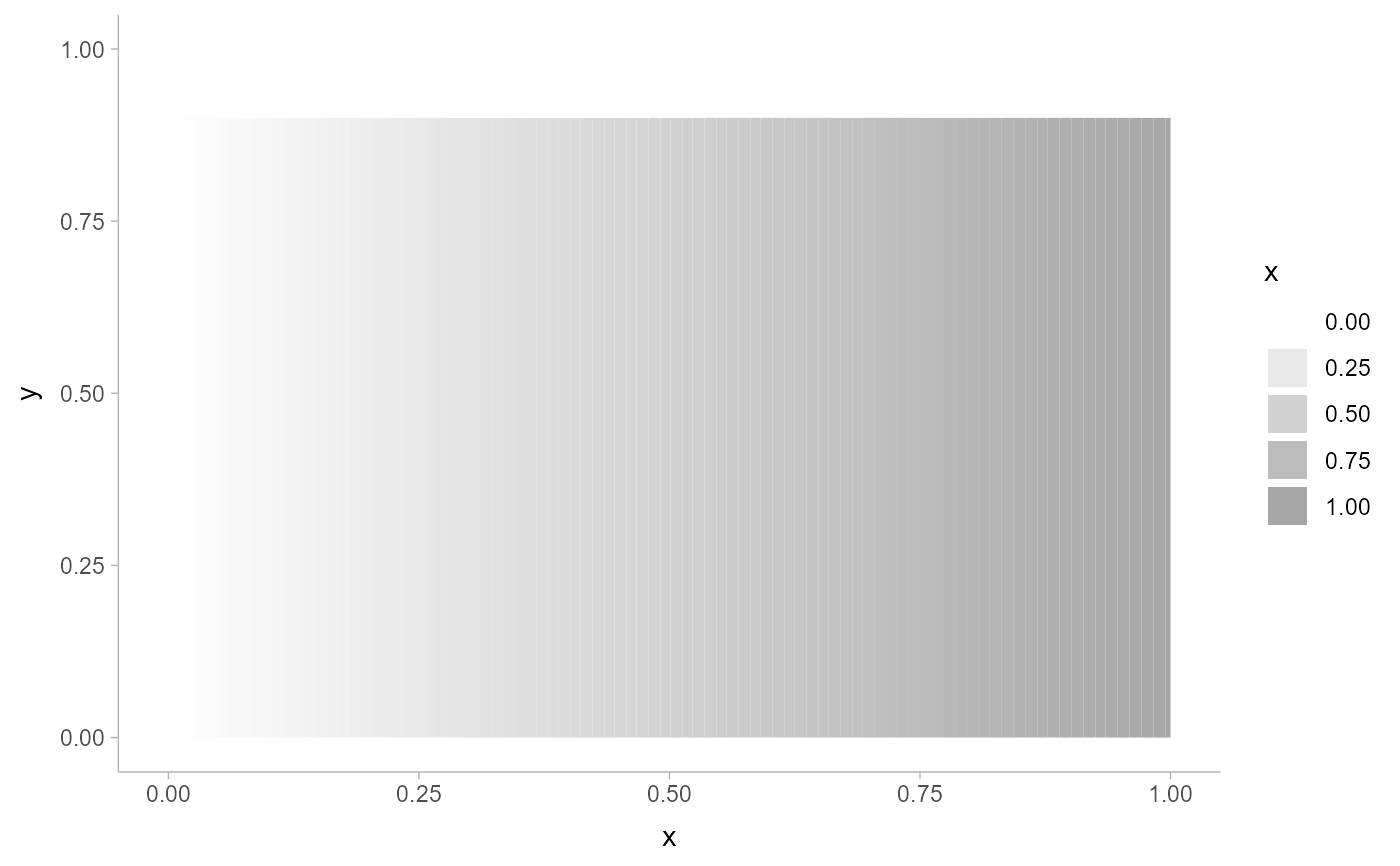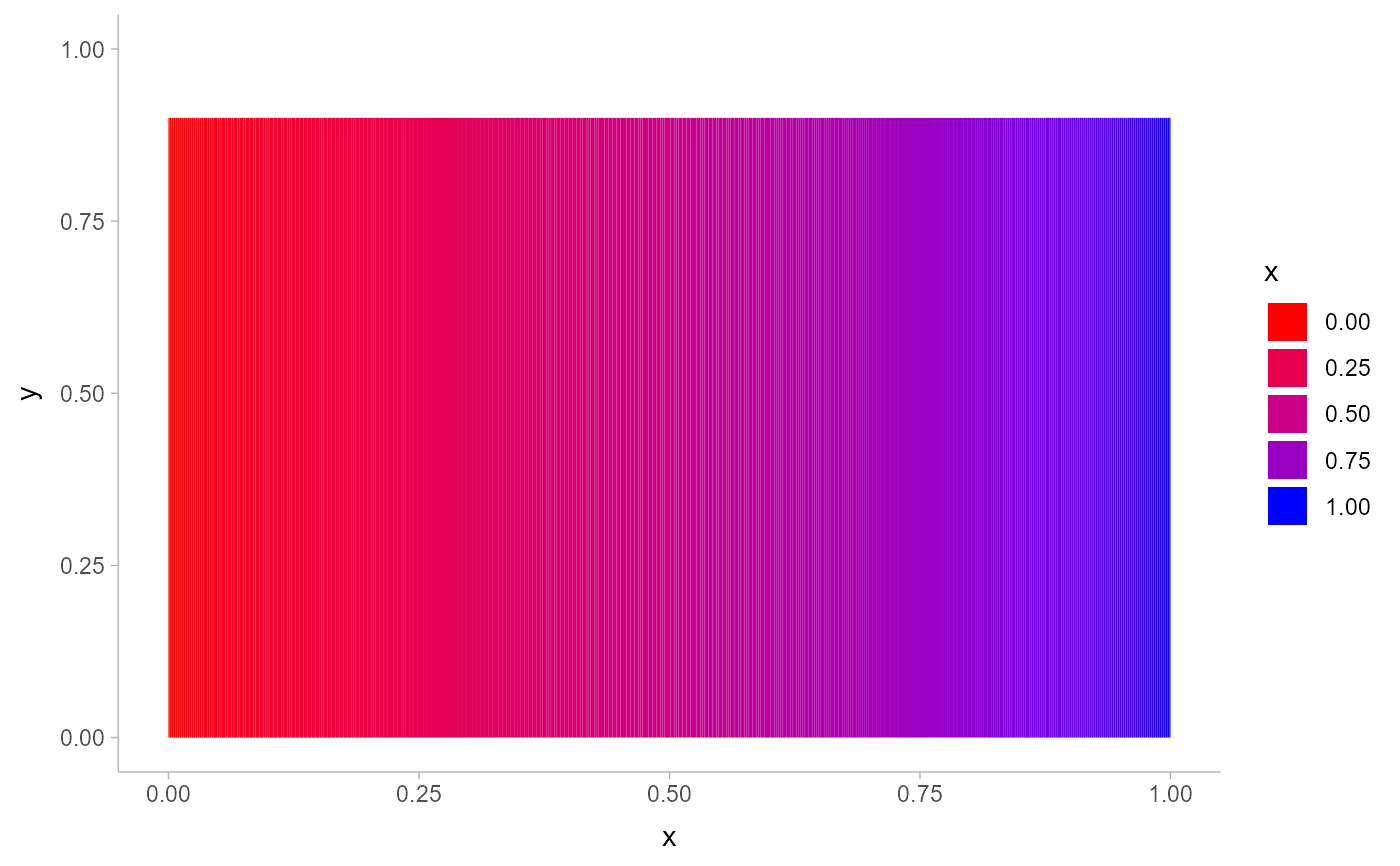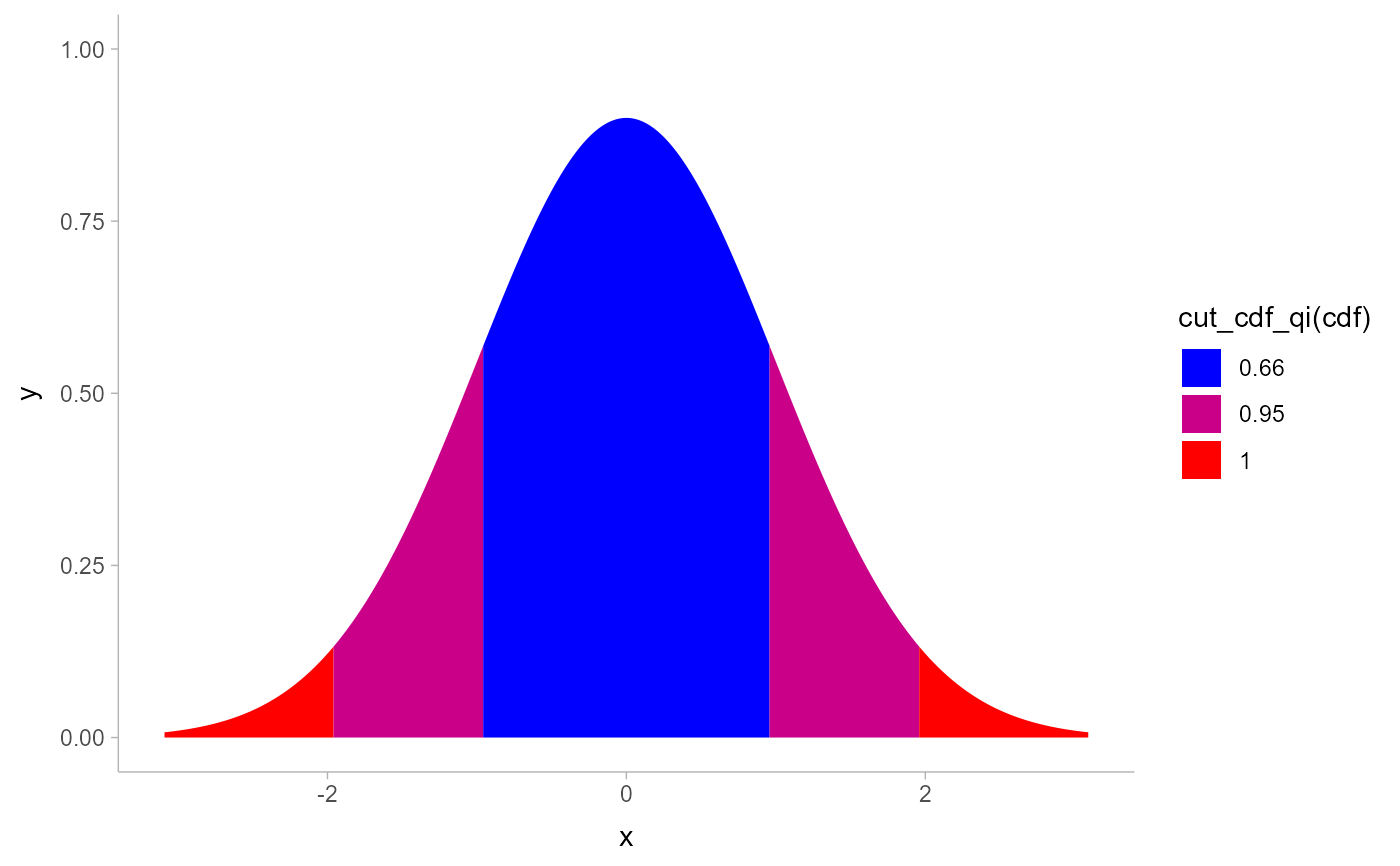This scale creates a secondary scale that modifies the fill or color scale of geoms that support it (geom_lineribbon() and geom_slabinterval()) to "ramp" from a secondary color (by default white) to the primary fill color (determined by the standard color or fill aesthetics).

scale_colour_ramp_continuous(
from = "white",
...,
limits = function(l) c(min(0, l[]), l[]),
range = c(0, 1),
guide = "legend",
aesthetics = "colour_ramp"
)

scale_color_ramp_continuous(
from = "white",
...,
limits = function(l) c(min(0, l[]), l[]),
range = c(0, 1),
guide = "legend",
aesthetics = "colour_ramp"
)

scale_colour_ramp_discrete(
from = "white",
...,
range = c(0.2, 1),
aesthetics = "colour_ramp"
)

scale_color_ramp_discrete(
from = "white",
...,
range = c(0.2, 1),
aesthetics = "colour_ramp"
)

scale_fill_ramp_continuous(..., aesthetics = "fill_ramp")

scale_fill_ramp_discrete(..., aesthetics = "fill_ramp")

## Arguments

from

The color to ramp from. Corresponds to 0 on the scale.

...

Arguments passed to underlying scale or guide functions. E.g. scale_colour_ramp_discrete(), passes arguments to discrete_scale(), scale_colour_ramp_continuous() passes arguments to continuous_scale(). See those functions for more details.

limits

One of:

• NULL to use the default scale range

• A numeric vector of length two providing limits of the scale. Use NA to refer to the existing minimum or maximum

• A function that accepts the existing (automatic) limits and returns new limits. Also accepts rlang lambda function notation. Note that setting limits on positional scales will remove data outside of the limits. If the purpose is to zoom, use the limit argument in the coordinate system (see coord_cartesian()).

range

a numeric vector of length 2 that specifies the minimum and maximum values after the scale transformation. These values should be between 0 (the from color) and 1 (the color determined by the fill aesthetic).

guide

A function used to create a guide or its name. For scale_colour_ramp_continuous() and scale_fill_ramp_continuous(), guide_rampbar() can be used to create gradient color bars. See guides() for information on other guides.

aesthetics

Names of aesthetics to set scales for.

## Value

A ggplot2::Scale representing a scale for the colour_ramp and/or fill_ramp

aesthetics for ggdist geoms. Can be added to a ggplot() object.

guide_rampbar()

Other ggdist scales: scale_side_mirrored(), scale_thickness, scales

Matthew Kay

## Examples


library(dplyr)
library(ggplot2)
library(distributional)

tibble(d = dist_uniform(0, 1)) %>%
ggplot(aes(y = 0, xdist = d)) +
stat_slab(aes(fill_ramp = after_stat(x)))tibble(d = dist_uniform(0, 1)) %>%
ggplot(aes(y = 0, xdist = d)) +
stat_slab(aes(fill_ramp = after_stat(x)), fill = "blue") +
scale_fill_ramp_continuous(from = "red")# you can invert the order of range to change the order of the blend
tibble(d = dist_normal(0, 1)) %>%
ggplot(aes(y = 0, xdist = d)) +
stat_slab(aes(fill_ramp = after_stat(cut_cdf_qi(cdf))), fill = "blue") +
scale_fill_ramp_discrete(from = "red", range = c(1, 0))